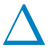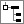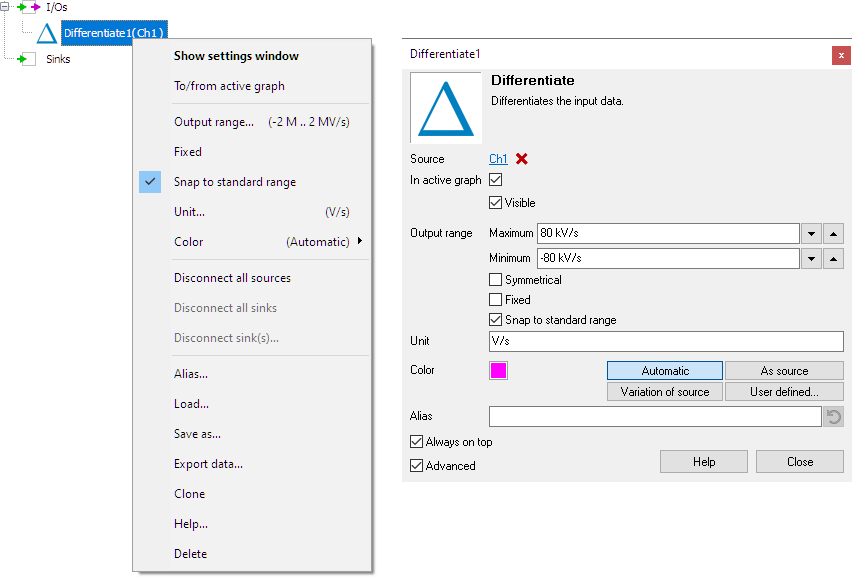# DifferentiateThe Differentiate I/O differentiates the source's data. The output is proportional to rate of change of the input. For example, if a source has the unit V, the output of the DIFF will have unit V/s. The output range can be changed and fixed to user defined values.

The typical application of the Differentiate I/O is to use it as part of larger, more complex mathematical operations.

Hint: The differentiate operation is very sensitive to noise, because noise in most cases has a higher frequency than the signal of interest. To minimize the effect of noise, add a low-pass filter processing block before or after the differentiate block. In case you are measuring a periodic triggered signal, you can also use an average I/O, which will give better results than filtering.

## Common properties and actions

To control the behavior of the Differentiate I/O, several properties are available. These can be accessed through a popup menu which is shown when the I/O is right clicked in the Object Tree. The properties can also be accessed through its settings window which is shown when the I/O is double clicked in the Object Tree. To open the Object tree, click theShow object tree button.By default, the settings window only shows the most used settings. When Advanced is ticked, the extended window with all settings is shown. See also the program settings.

### Related information

#### Gain / Offset

The Gain / Offset I/O multiplies a signal with a constant gain factor and adds a constant offset.

The Add / Subtract I/O adds or subtracts data of two or more sources.

#### Multiply / Divide

The Multiply / Divide I/O multiplies or divides data of two ore more sources.

#### Sqrt

The SQRT I/O calculates the square root of each sample of the source's data.

#### ABS

The ABS I/O takes the absolute value of each sample of the source's data.

#### Integrate

The Integrate I/O integrates its source's data.

#### Logarithm

The Logarithm I/O calculates the logarithm of the source's data.

#### Average

The Average I/O averages successive measurements and outputs the average of these measurements.

#### Low pass filter

The Low pass filter I/O filters the source's data using a first order low pass filter.